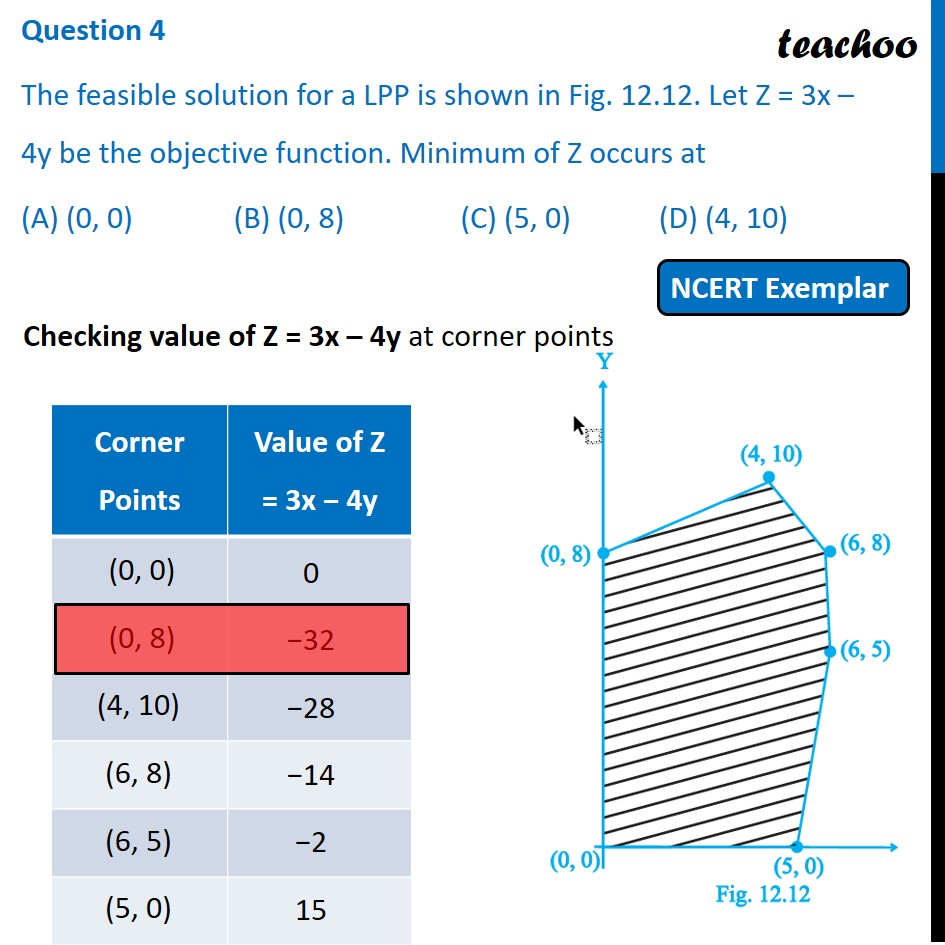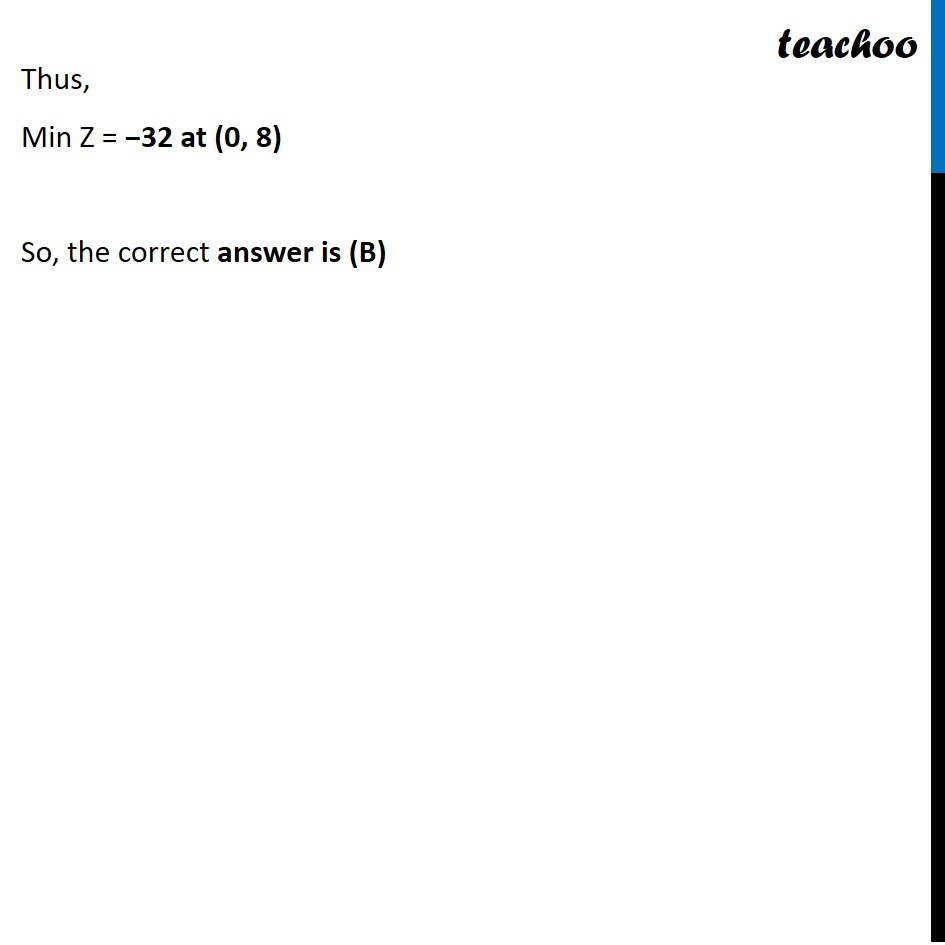NCERT Exemplar - MCQs

Chapter 12 Class 12 Linear Programming
Serial order wise

## (C) (5, 0)                               (D) (4, 10)

This question is similar to Ex 12.2, 11 - Chapter 12 Class 12 - Linear ProgrammingLearn in your speed, with individual attention - Teachoo Maths 1-on-1 Class

### Transcript

Question 4 The feasible solution for a LPP is shown in Fig. 12.12. Let Z = 3x – 4y be the objective function. Minimum of Z occurs at (A) (0, 0) (B) (0, 8) (C) (5, 0) (D) (4, 10) Checking value of Z = 3x – 4y at corner points Thus, Min Z = −32 at (0, 8) So, the correct answer is (B)